# Ionic Equations Reactions Equations Molecular equations show the

• Slides: 31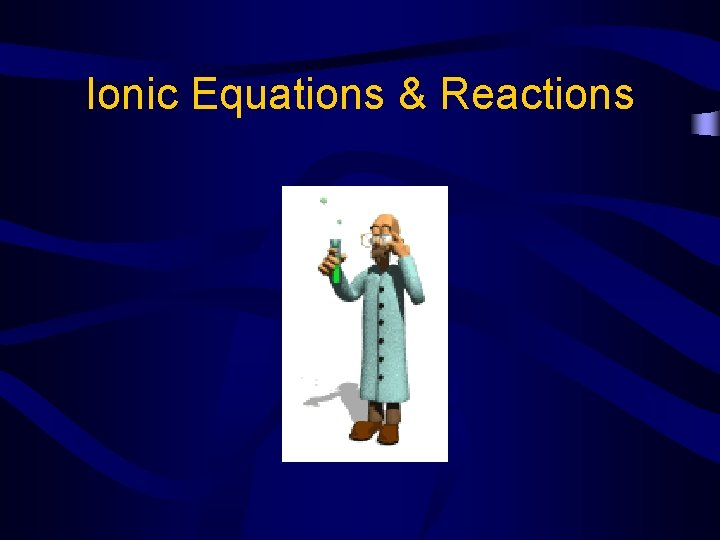Ionic Equations & Reactions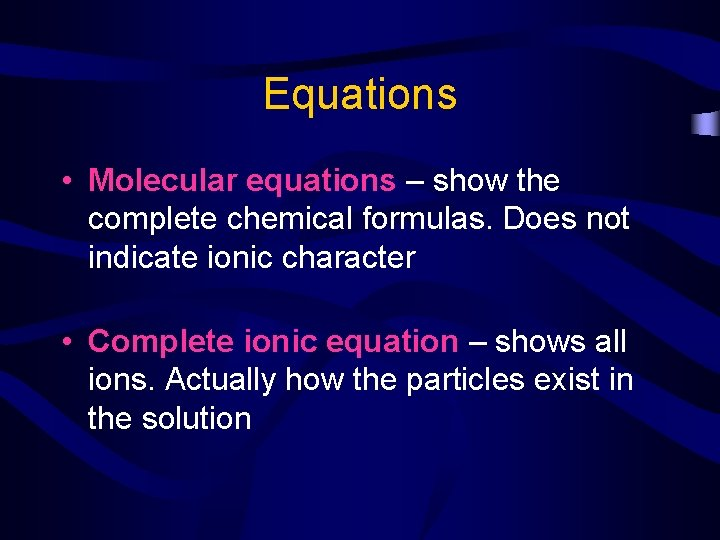Equations • Molecular equations – show the complete chemical formulas. Does not indicate ionic character • Complete ionic equation – shows all ions. Actually how the particles exist in the solutionSteps for Writing Ionic Equations 1. Write the balances molecular equation (balanced chemical equation) 2. Break every thing down into its ions EXCEPT the solid, gas, water, or weak electrolyte (complete ionic equation) 3. Cross out everything that is the same on both sides (spectator ions) 4. Write what is left (net ionic equation)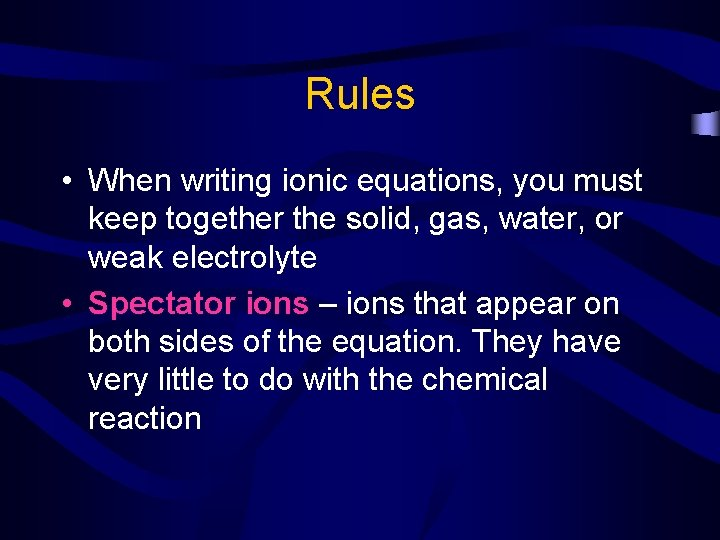Rules • When writing ionic equations, you must keep together the solid, gas, water, or weak electrolyte • Spectator ions – ions that appear on both sides of the equation. They have very little to do with the chemical reaction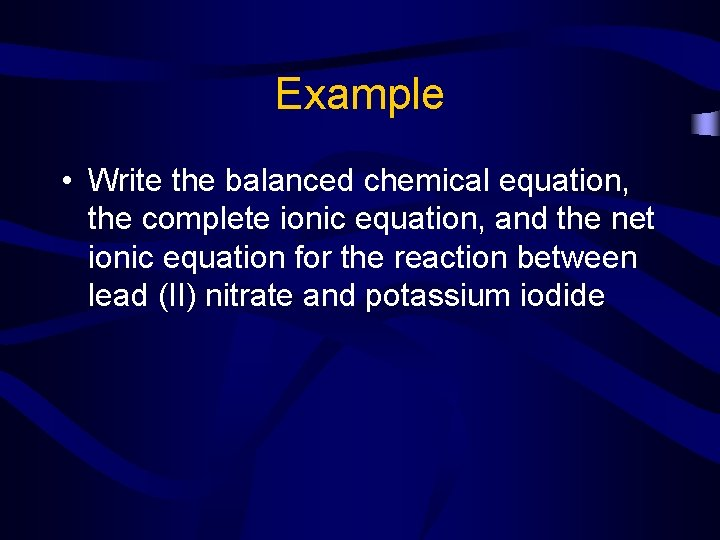Example • Write the balanced chemical equation, the complete ionic equation, and the net ionic equation for the reaction between lead (II) nitrate and potassium iodide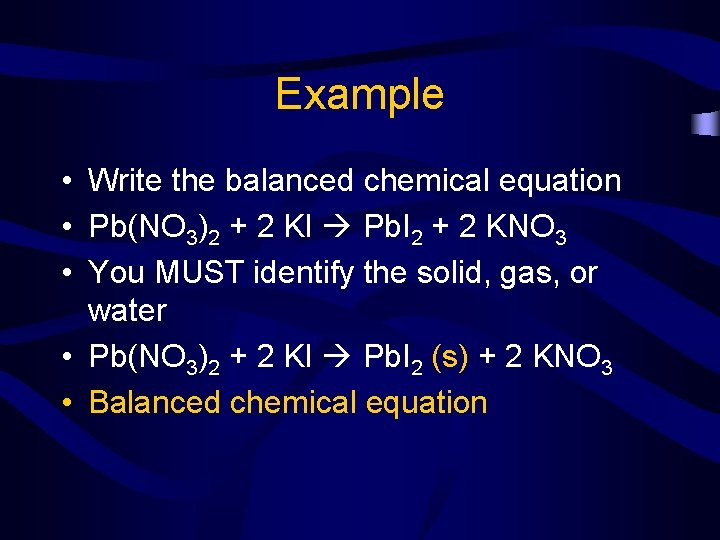Example • Write the balanced chemical equation • Pb(NO 3)2 + 2 KI Pb. I 2 + 2 KNO 3 • You MUST identify the solid, gas, or water • Pb(NO 3)2 + 2 KI Pb. I 2 (s) + 2 KNO 3 • Balanced chemical equationExample • Now break every thing except the solid, gas, or water into its ions • Remember ions are things with charges • Everything will be broken down into one positive charge and one negative chargeExample • Pb(NO 3)2 + 2 KI Pb. I 2 (s) + 2 KNO 3 • Pb+2 + 2 NO 3 -1 + 2 K+1 + 2 I -1 Pb. I 2 (s) + 2 K+1 + 2 NO 3 -1 • Complete ionic Equation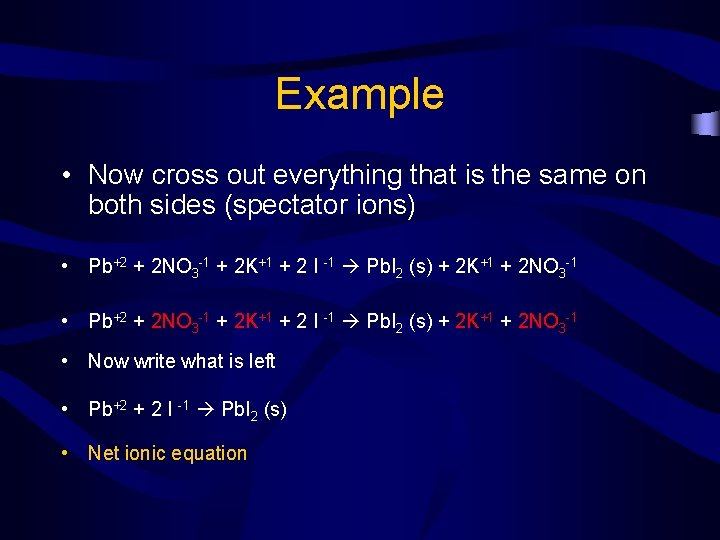Example • Now cross out everything that is the same on both sides (spectator ions) • Pb+2 + 2 NO 3 -1 + 2 K+1 + 2 I -1 Pb. I 2 (s) + 2 K+1 + 2 NO 3 -1 • Now write what is left • Pb+2 + 2 I -1 Pb. I 2 (s) • Net ionic equation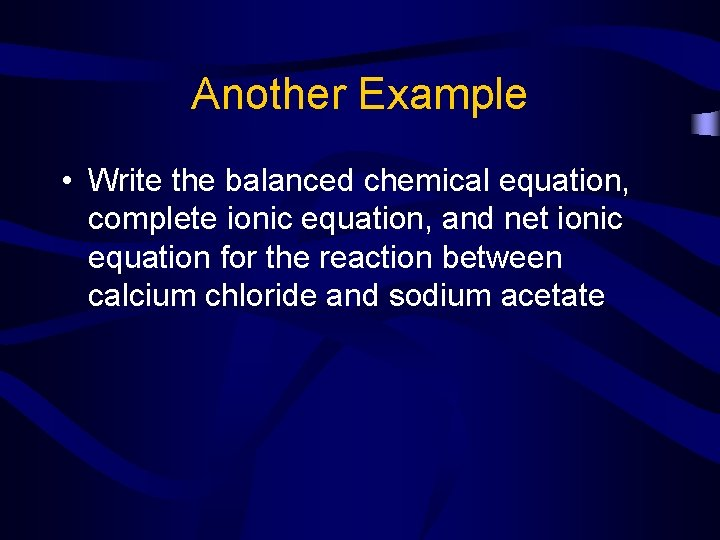Another Example • Write the balanced chemical equation, complete ionic equation, and net ionic equation for the reaction between calcium chloride and sodium acetateAnother Example • Balanced chemical equation • Ca. Cl 2 + Na 2 CO 3 Ca. CO 3 (s) + 2 Na. Cl • Complete ionic equation • Ca+2 + 2 Cl -2 + 2 Na +1 + CO 3 -2 Ca. CO 3 (s) + 2 Na +1 + 2 Cl -1 • Net Ionic Equation • Ca+2 + 2 Cl -2 + 2 Na +1 + CO 3 -2 Ca. CO 3 (s) + 2 Na +1 + 2 Cl -1 • Ca+2 + CO 3 -2 Ca. CO 3 (s)What if water is formed? • Write the balanced chemical equation, complete ionic equation, and net ionic equation for the reaction between Calcium hydroxide and nitric acid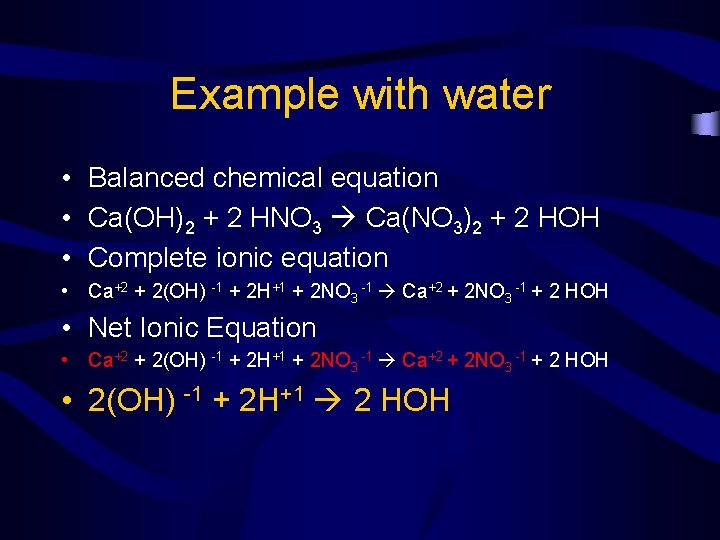Example with water • Balanced chemical equation • Ca(OH)2 + 2 HNO 3 Ca(NO 3)2 + 2 HOH • Complete ionic equation • Ca+2 + 2(OH) -1 + 2 H+1 + 2 NO 3 -1 Ca+2 + 2 NO 3 -1 + 2 HOH • Net Ionic Equation • Ca+2 + 2(OH) -1 + 2 H+1 + 2 NO 3 -1 Ca+2 + 2 NO 3 -1 + 2 HOH • 2(OH) -1 + 2 H+1 2 HOH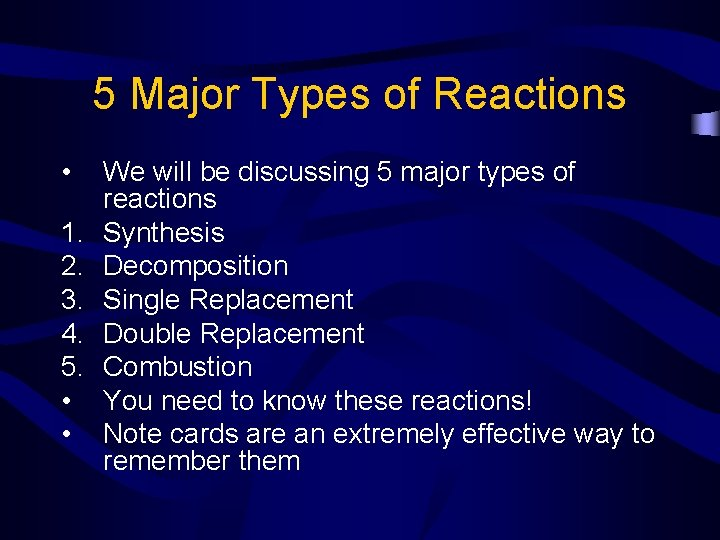5 Major Types of Reactions • 1. 2. 3. 4. 5. • • We will be discussing 5 major types of reactions Synthesis Decomposition Single Replacement Double Replacement Combustion You need to know these reactions! Note cards are an extremely effective way to remember them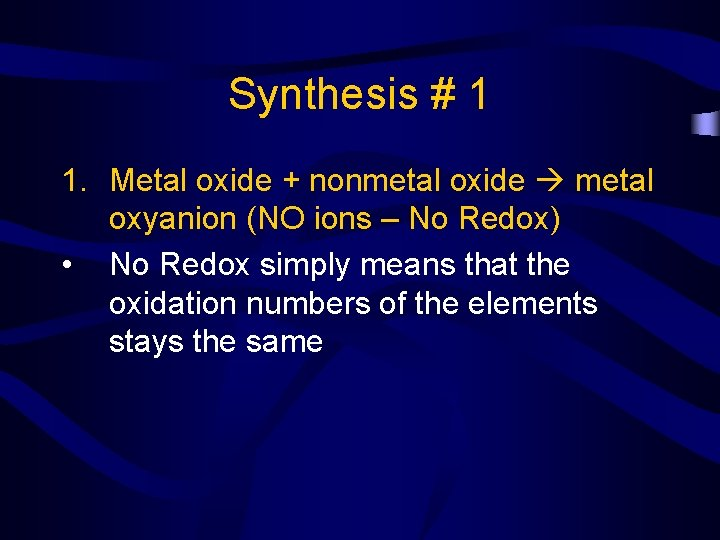Synthesis # 1 1. Metal oxide + nonmetal oxide metal oxyanion (NO ions – No Redox) • No Redox simply means that the oxidation numbers of the elements stays the same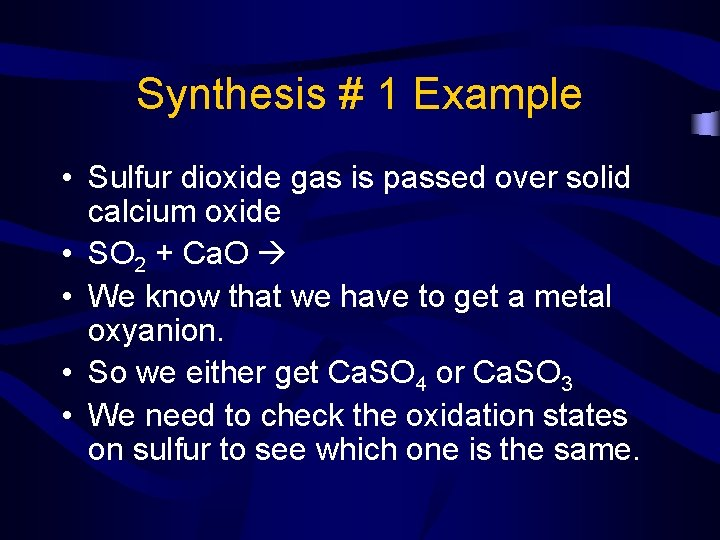Synthesis # 1 Example • Sulfur dioxide gas is passed over solid calcium oxide • SO 2 + Ca. O • We know that we have to get a metal oxyanion. • So we either get Ca. SO 4 or Ca. SO 3 • We need to check the oxidation states on sulfur to see which one is the same.Synthesis # 1 Example • In SO 2, the oxidation number of O is -2 • So the oxidation number of S must be +4 • Our product choices are Ca. SO 3 or Ca. SO 4 • In Ca. SO 3…S has an oxidation # of +4 • In Ca. SO 4…S has an oxidation # of +6 • Therefore the product must be Ca. SO 3 • SO 2 + Ca. O Ca. SO 3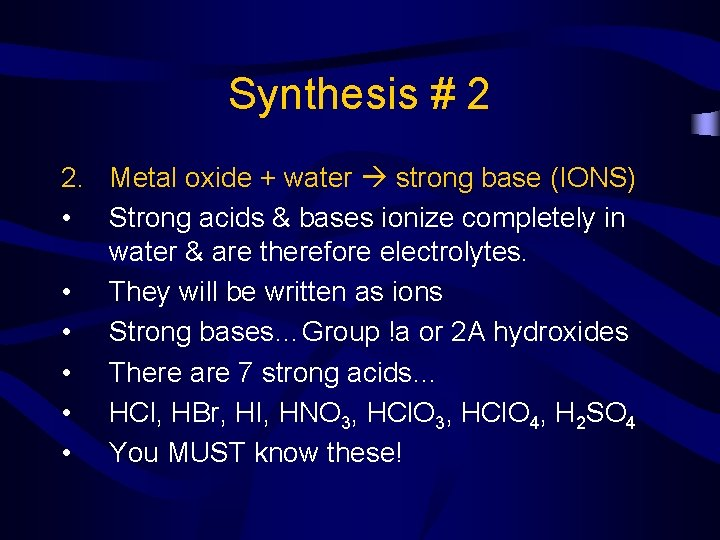Synthesis # 2 2. Metal oxide + water strong base (IONS) • Strong acids & bases ionize completely in water & are therefore electrolytes. • They will be written as ions • Strong bases…Group !a or 2 A hydroxides • There are 7 strong acids… • HCl, HBr, HI, HNO 3, HCl. O 4, H 2 SO 4 • You MUST know these!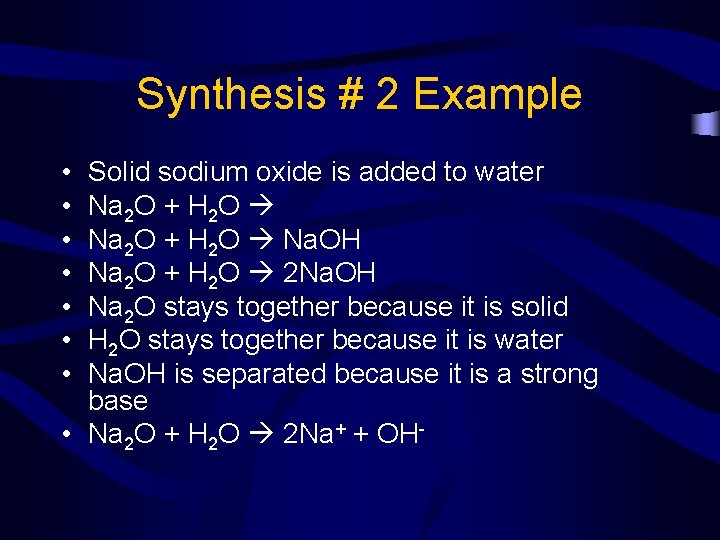Synthesis # 2 Example • • Solid sodium oxide is added to water Na 2 O + H 2 O Na. OH Na 2 O + H 2 O 2 Na. OH Na 2 O stays together because it is solid H 2 O stays together because it is water Na. OH is separated because it is a strong base • Na 2 O + H 2 O 2 Na+ + OH-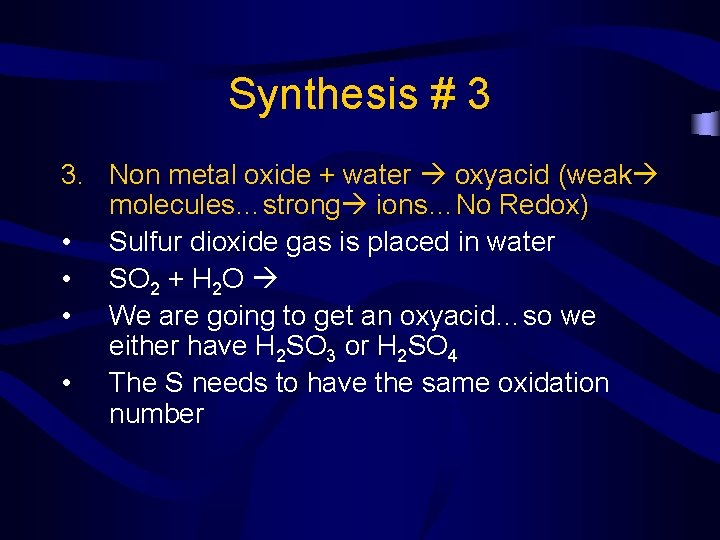Synthesis # 3 3. Non metal oxide + water oxyacid (weak molecules…strong ions…No Redox) • Sulfur dioxide gas is placed in water • SO 2 + H 2 O • We are going to get an oxyacid…so we either have H 2 SO 3 or H 2 SO 4 • The S needs to have the same oxidation numberSynthesis # 3 Example • In SO 2, O has an oxidation # of -2…so S has an oxidation # of +4 • In H 2 SO 3…S has an oxidation # of +4 • In H 2 SO 4…S has an oxidation # of +6 • Therefore we will get In H 2 SO 3 • SO 2 + H 2 O H 2 SO 3 • Since H 2 SO 3 is a weak acid…we will keep it togetherSynthesis # 4 4. Metal + nonmetal salt (NO ions) • A salt is just an ionic compound ( a positive charge & a negative charge) • Magnesium metal is combusted in nitrogen gas • Mg + N 2 Mg 3 N 2 • 3 Mg + N 2 Mg 3 N 2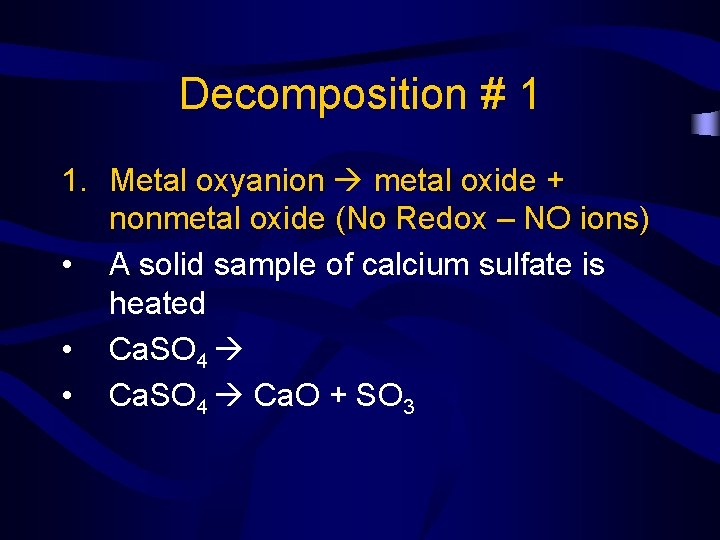Decomposition # 1 1. Metal oxyanion metal oxide + nonmetal oxide (No Redox – NO ions) • A solid sample of calcium sulfate is heated • Ca. SO 4 Ca. O + SO 3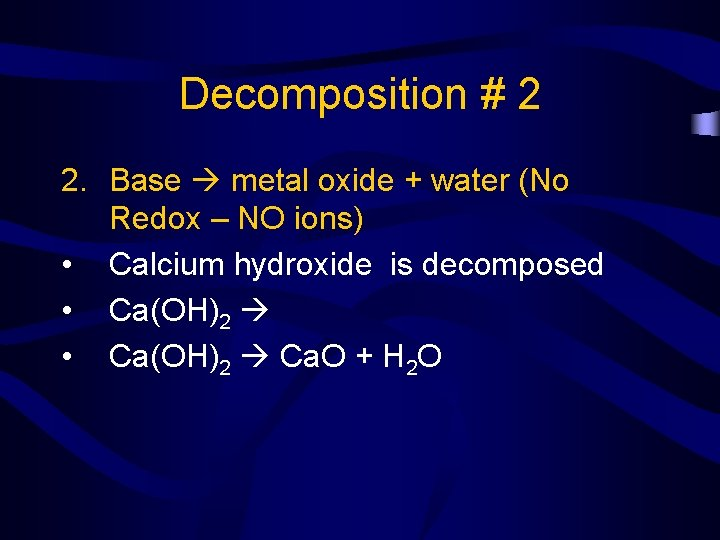Decomposition # 2 2. Base metal oxide + water (No Redox – NO ions) • Calcium hydroxide is decomposed • Ca(OH)2 Ca. O + H 2 O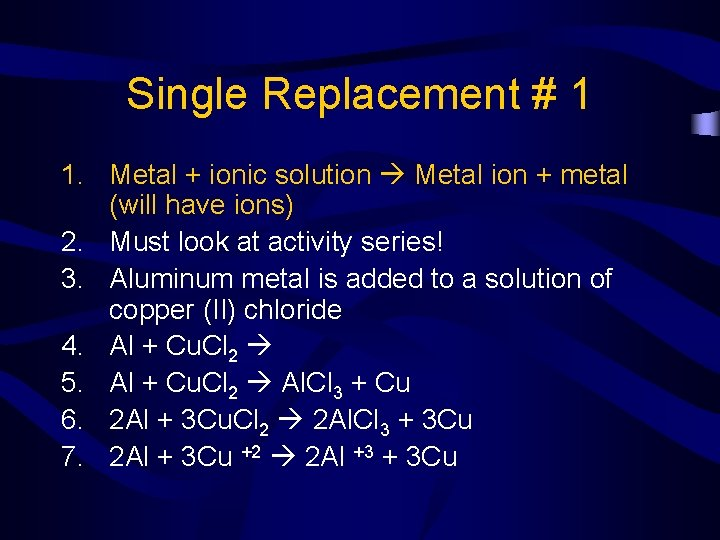Single Replacement # 1 1. Metal + ionic solution Metal ion + metal (will have ions) 2. Must look at activity series! 3. Aluminum metal is added to a solution of copper (II) chloride 4. Al + Cu. Cl 2 5. Al + Cu. Cl 2 Al. Cl 3 + Cu 6. 2 Al + 3 Cu. Cl 2 2 Al. Cl 3 + 3 Cu 7. 2 Al + 3 Cu +2 2 Al +3 + 3 CuSingle Replacement # 2 2. Active metal (Group 1 A, Ba, Ca, Sr) + water H 2 + strong base (IONS) • Sodium is placed in water • Na + H 2 O H 2 + Na. OH • 2 Na + 2 H 2 O H 2 + 2 Na+ + 2 OH-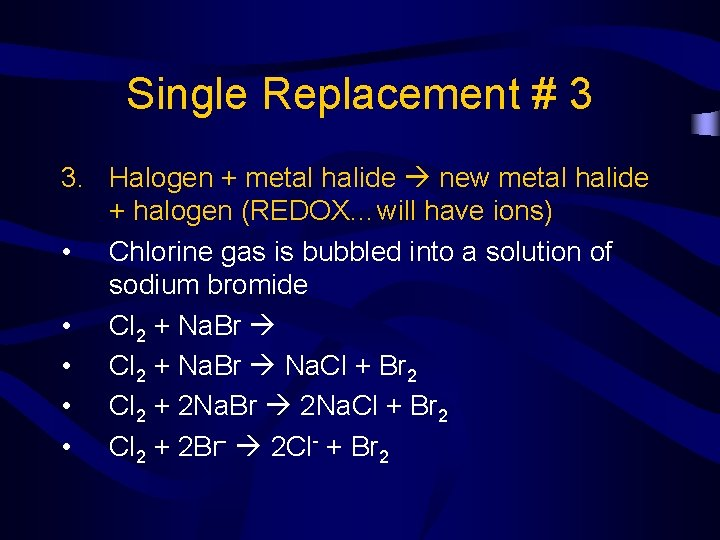Single Replacement # 3 3. Halogen + metal halide new metal halide + halogen (REDOX…will have ions) • Chlorine gas is bubbled into a solution of sodium bromide • Cl 2 + Na. Br Na. Cl + Br 2 • Cl 2 + 2 Na. Br 2 Na. Cl + Br 2 • Cl 2 + 2 Br- 2 Cl- + Br 2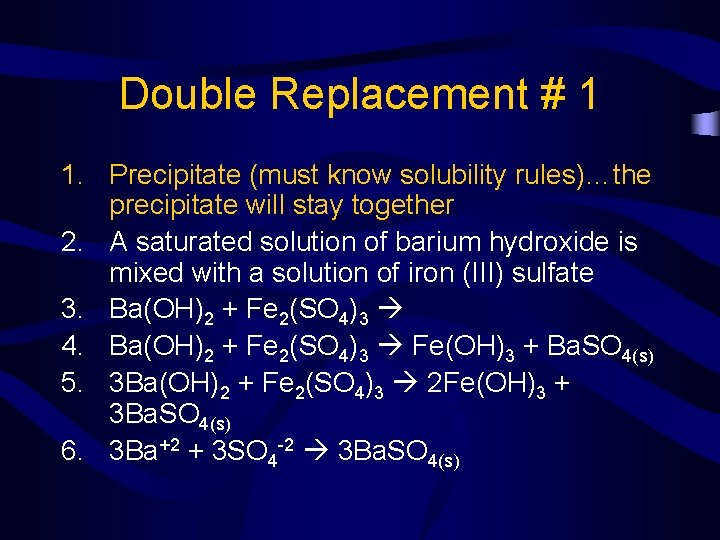Double Replacement # 1 1. Precipitate (must know solubility rules)…the precipitate will stay together 2. A saturated solution of barium hydroxide is mixed with a solution of iron (III) sulfate 3. Ba(OH)2 + Fe 2(SO 4)3 4. Ba(OH)2 + Fe 2(SO 4)3 Fe(OH)3 + Ba. SO 4(s) 5. 3 Ba(OH)2 + Fe 2(SO 4)3 2 Fe(OH)3 + 3 Ba. SO 4(s) 6. 3 Ba+2 + 3 SO 4 -2 3 Ba. SO 4(s)Double Replacement # 2 2. Formation of a gas (acid + sulfide, carbonate, or bicarbonate) • Hydrobromic acid is added to a solution of potassium bicarbonate • HBr + KHCO 3 H 2 CO 3 + KBr • H 2 CO 3 ALWAYS breaks down into CO 2 + H 2 O • HBr + KHCO 3 CO 2 + H 2 O + KBr • H+ + HCO 3 - CO 2 + H 2 O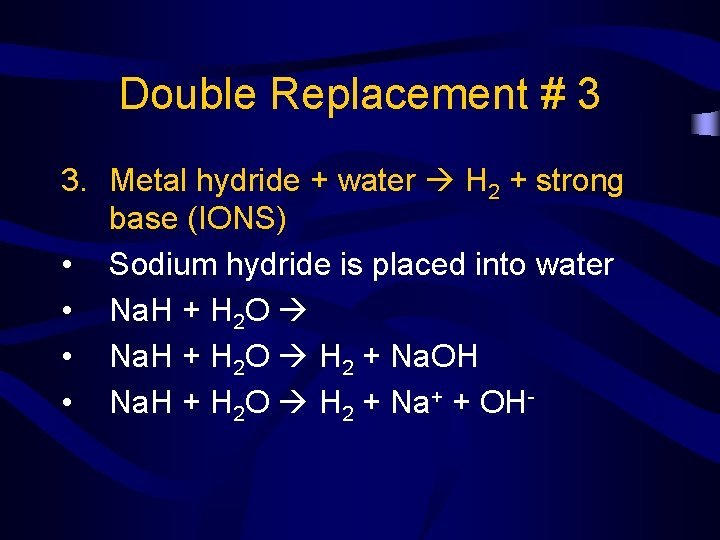Double Replacement # 3 3. Metal hydride + water H 2 + strong base (IONS) • Sodium hydride is placed into water • Na. H + H 2 O H 2 + Na. OH • Na. H + H 2 O H 2 + Na+ + OH-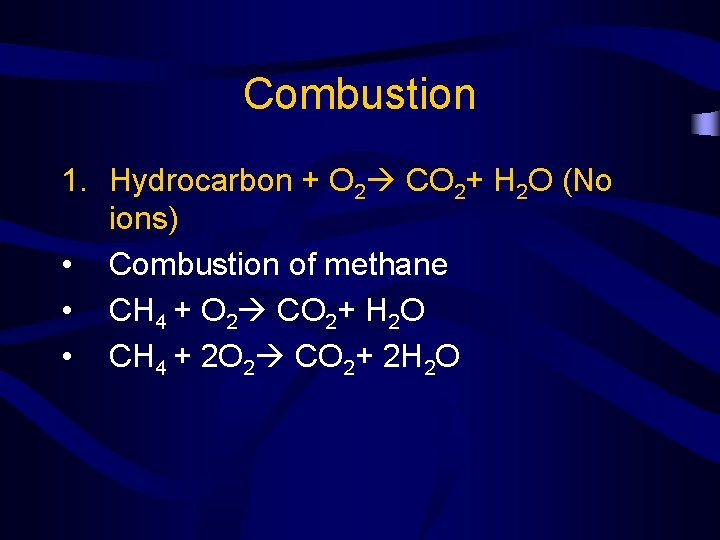Combustion 1. Hydrocarbon + O 2 CO 2+ H 2 O (No ions) • Combustion of methane • CH 4 + O 2 CO 2+ H 2 O • CH 4 + 2 O 2 CO 2+ 2 H 2 O